-->

# Electrostatic Type Instruments Working Principle,Construction,Torque Equation And Extending Range

## Working principle, construction , torque equation and extending range of electrostatic type instruments

Hello readers, In this post we are going to discuss about construction principle and torque equation of electrostatic type instruments.

### Working principle of electrostatic type instruments:

Working principle of electrostatic type instruments is electrostatic effect.

#### What is meant by electrostatic induction??

To understand clearly about electrostatic effect see the below circuit.

1.Here the two plates are being charged by a high voltage battery.

2. Due to this one of the plate gets positive charge and the other plate gets negative charge.

3. Here the deflecting torque is produced by this static electrical field due to attraction present between these opposite charges.

4. The plates move because of the electrostatic force(attraction between plates) that has been produced because of this induced charges.

This effect is called electrostatic effect.

### Construction of electrostatic type instruments:

1. Linear type electrostatic instruments.

2. Rotatory type electrostatic instruments.

### Linear type electrostatic instruments:

1. Here one of the plates is fixed and the other plate is movable and these plates are charged as shown in the above circuit. So one of the plate gets positive charge and the other plates gets negative charge. Due to this there will be force of attraction between the plates so the movable plate moves towards the fixed plate until movable plate gains maximum amount of electrostatic energy. Fixing pointer to the movable plate we can measure the voltage. These are called linear type electrostatic instruments.

### Rotatory type electrostatic instruments:

2. Here we have a rotatory plate. Due this movement of rotatory plate there may be force of attraction or repulsion between the plates. These are called rotatory type electrostatic instruments.

### Torque equation of electrostatic type instruments:

Now let us see torque equation of both linear type electrostatic instruments and rotatory type electrostatic instruments.

### Torque equation of linear type electrostatic instruments:

Let us see in detail about torque equation of liner type electrostatic instruments.

Observe the following diagram.

1.Here plate A is fixed and it is positively charged and plate B is movable and it is negatively charged.

2. As the forces are opposite we have attraction between plates. So there will be linear motion between these plates.

3. As there is force between these plates at equilibrium electrostatic force will be equal to spring force.

4.Now electrostatic energy stored in the plate is given by,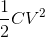5.Now let us increase the voltage by a small amount let it be dv due to this there will be displacement of plate let the displacement be dx. So work done against the spring force due to displacement of  plate B be F.dx.  Relation between current and applied voltage is given by,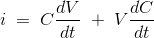6. Now the input energy from this value of electric current is given by,

7. Now the change in this stored energy is given by,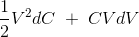8. Now apply principle of energy conservation by neglecting the higher order terms in the expression.

Input energy to the system = increase in the stored energy of the system + mechanical work done by the system.

By substituting all the values we get,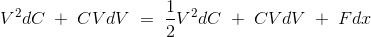Now the equation of force from the above equation is given by,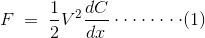### Torque equation of rotatory type electrostatic instruments:

Let us see in detail about torque equation of rotatory type electrostatic instruments.

Observe the following diagram.

1. By replacing F, dx in equation (1)  by Td , dA respectively we get deflecting torque of rotary type electrostatic instruments.

2. So the deflecting torque is given by,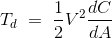3.At steady state we have controlling torque is given by, Tc = K × A. Where A is the deflection and it is given by,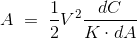As the deflection is directly proportional to square of voltage we have non- uniform scale.

Hence we have derived  torque equation of  electrostatic type instruments i.e for liner type electrostatic instruments and  rotatory type electrostatic instruments.

Generally electrostatic type instruments are used for measuring high voltages.

The main advantage of using electrostatic type instruments as voltmeters is we can extend the range of voltage that is to be measured.

### Methods to extend the range of voltage to be measured for electrostatic instruments:

1. Resistance potential dividers.

2.Capacitor multiplier technique.

### Resistance potential dividers to extend the range of voltage to be measured for electrostatic instruments:

Now let us see how to extend the range of voltage to be measured by using resistance potential dividers.

To understand it see the below circuit.

### Circuit diagram of resistance potential dividers to extend the range of voltage to be measured for electrostatic instruments:

The following diagram shows the circuit to extend the range of voltage to be measured by
electrostatic instruments using resistance potential dividers.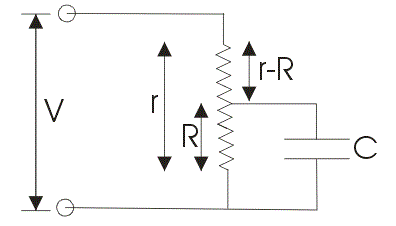### Procedure to extend the range of voltage to be measured by electrostatic instruments using resistance potential dividers:

1. Across r which is total resistance apply the voltage which is to be measured.

2. Across R which is a part of total resistance r connect an electrostatic capacitor.

3.Make one assumption that the capacitor which is connected is having infinite leakage resistance in case if we apply dc voltage. Here the multiplying factor is ratio of resistances i.e, r/R. Multiplying factor in ac case is same as dc case.

### Capacitor multiplier technique to extend the range of voltage to be measured for electrostatic instruments:

Now let us see how to extend the range of voltage to be measured by electrostatic instruments
using capacitor multiplier technique.

To understand it see the below circuit.

### Circuit diagram of capacitor multiplier technique to extend the range of voltage to be measured for electrostatic instruments:

The following diagram shows the circuit to extend the range of voltage to be measured by electrostatic instruments using capacitor multiplier technique.

### Procedure to extend the range of voltage to be measured by electrostatic instruments  using capacitor multiplier technique:

Let us calculate the multiplying factor.

1. From diagram we have series combination of capacitors. The equivalent capacitance is given by

2. Voltmeter impedance is given by Z1 = 1/jωC1 . Now total impedance is given by,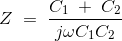3.Multiplying factor is given by,

Z/Z1 = 1 + C2 / C1.

In this way we can extend the range of voltage to be measured by electrostatic instruments with the help of  resistance potential dividers and capacitor multiplier technique.

### Advantages of electrostatic type instruments:

1. As the deflection torque is directly proportional to square of voltage we can measure both a.c and d.c voltages by using electrostatic type instruments.

2.High values of voltage can be measured  using electrostatic type instruments.

3. Current drawn by electrostatic type instruments  is low so power consumption of electrostatic type instruments is low.

### Disadvantages of electrostatic type instruments:

1.Electrostatic type instruments have non uniform scale.

2.Electrostatic type instruments are larger in size.

3.Electrostatic type instruments are costlier compared to other type of instruments.

4.Various operating forces present in electrostatic type instruments are small in magnitude.

Today we have learnt working principle, construction , torque equation and extending range of electrostatic type instruments.